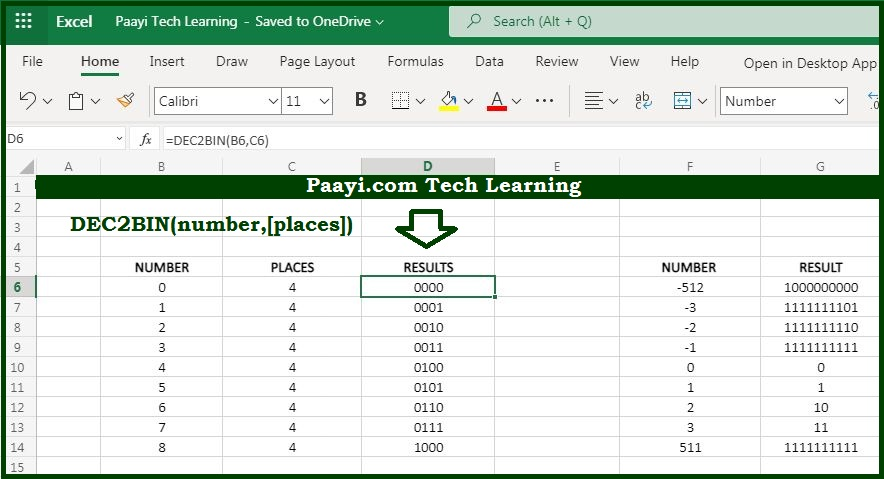# Learn How to Use Microsoft Excel DEC2BIN Function

Written by | 0 Comments | 762 Views

In this article, you will learn how to use the Microsoft Excel DEC2BIN function and its prime function in Microsoft Excel. You will also get to know the Microsoft Excel DEC2BIN function return value and syntax with the help of some examples.

Microsoft Excel DEC2BIN Function

The prime function of the Microsoft Excel DEC2BIN function is to convert decimal numbers to their binary equivalent. That means with the help of the DEC2BIN function you can able to convert a decimal number that represents its binary equivalent.

Return Value of DEC2BIN Function

The return value will be the binary number.

Syntax of DEC2BIN Function

=DEC2BIN(number, [places])

Where the arguments:

• number: It is the decimal number that you want to convert into binary equivalent.
• places: Holds the resulting binary number with zeros up to the specified number of digits. In case omitted returns the least number of characters required to represent the number (optional).

## How to Use Microsoft Excel DEC2BIN Function?As we know that DEC2BIN function used to convert the specified decimal number to its binary counterpart. Just make sure that the input must be a valid number within the range of -512 to 511.